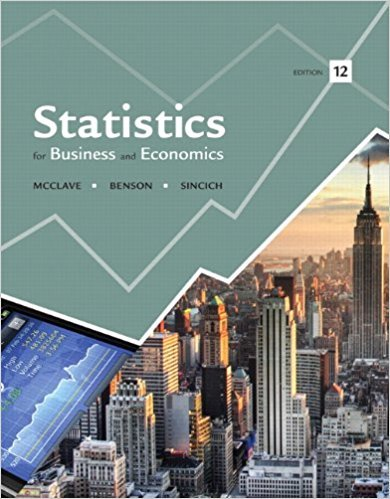×
Log in to StudySoup
Get Full Access to Statistics For Business And Economics - 12 Edition - Chapter 6 - Problem 5e
Join StudySoup for FREE
Get Full Access to Statistics For Business And Economics - 12 Edition - Chapter 6 - Problem 5e

Already have an account? Login here
×
Reset your password

# A random sample of 70 observations from a normallyISBN: 9780321826237 51

## Solution for problem 5E Chapter 6

Statistics for Business and Economics | 12th Edition

• Textbook Solutions
• 2901 Step-by-step solutions solved by professors and subject experts
• Get 24/7 help from StudySoup virtual teaching assistantsStatistics for Business and Economics | 12th Edition

4 5 1 413 Reviews
26
1
Problem 5E

Problem 5E

A random sample of 70 observations from a normally distributed population possesses a sample mean equal to 26.2 and a sample standard deviation equal to 4.1.

a. Find an approximate 95% confidence interval for μ.

b. What do you mean when you say that a confidence coefficient is .95?

c. Find an approximate 99% confidence interval for μ.

d. What happens to the width of a confidence interval as the value of the confidence coefficient is increased while the sample size is held fixed?

e. Would your confidence intervals of parts a and c be valid if the distribution of the original population was not normal? Explain.

Step-by-Step Solution:

Answer

Step 1 of 5

(a)

Given a random sample ofobservations which follows a normally distributed population.

Given sample meanand sample standard deviationWe are asked to find an approximateconfidence interval forLetbe a large sample of sizefrom a population with meanand standard deviationthen a levelconfidence interval foris……………..(1)

Given sample mean,  sample standard deviationandSince we want 95% confidence interval, then a level isandThenforis, henceHence 95% confidence interval forisHence  a 95% confidence interval foris.

Step 2 of 5

Step 3 of 5

##### ISBN: 9780321826237

Unlock Textbook Solution

Enter your email below to unlock your verified solution to:

A random sample of 70 observations from a normally